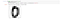# Recognizing Handwritten Digits

Data analysis is not limited to numbers and strings, because images and sounds can also be analyzed and classified.

In this blog,I have performed data analysis on recognizing Handwritten Digits with scikit-learn.

Recognizing handwritten text is a problem that can be traced back to the first automatic machines that needed to recognize individual characters in handwritten documents. Think about, for example, the ZIP codes on letters at the post office and the automation needed to recognize these five digits. Perfect recognition of these codes is necessary in order to sort mail automatically and efficiently. Included among the other applications that may come to mind is OCR (Optical Character Recognition) software. OCR software must read handwritten text, or pages of printed books, for general electronic documents in which each character is well defined. But the problem of handwriting recognition goes farther back in time, more precisely to the early 20th Century (1920s), when Emanuel Goldberg (1881–1970) began his studies regarding this issue and suggested that a statistical approach would be an optimal choice. To address this issue in Python, the scikit-learn library provides a good example to better understand this technique, the issues involved, and the possibility of making predictions.

Here,the data to be analyzed is closely related to numerical values or strings, but can also involve images and sounds. The problem we have to face here involves predicting a numeric value, and then reading and interpreting an image that uses a handwritten font.

An estimator that is useful in this case is sklearn.svm.SVC, which uses the technique of Support Vector Classification (SVC). Thus, we have to import the svm module of the scikit-learn library. We can create an estimator of SVC type and then choose an initial setting, assigning the values C and gamma generic values. These values can then be adjusted in a different way during the course of the analysis.

Tuning parameters: Regularization, Gamma.

Regularization :

The Regularization parameter (often termed as C parameter in python’s sklearn library) tells the SVM optimization how much we want to avoid misclassifying each training example.

For large values of C, the optimization will choose a smaller-margin hyperplane if that hyperplane does a better job of getting all the training points classified correctly. Conversely, a very small value of C will cause the optimizer to look for a larger-margin separating hyperplane, even if that hyperplane misclassifies more points.

Gamma :

The gamma parameter defines how far the influence of a single training example reaches, with low values meaning ‘far’ and high values meaning ‘close’. In other words, with low gamma, points far away from plausible seperation line are considered in calculation for the seperation line. Where as high gamma means the points close to plausible line are considered in calculation.

The Digits Dataset The scikit-learn library provides numerous datasets that are useful for testing many problems of data analysis and prediction of the results. Also in this case there is a dataset of images called Digits. This dataset consists of 1,797 images that are 8x8 pixels in size.One of 1,797 handwritten number images that make up the dataset digit

Now that we have loaded the Digits datasets into your notebook and have defined an SVC estimator, you can start learning. Once you define a predictive model, you must instruct it with a training set, which is a set of data in which you already know the belonging class. Given the large quantity of elements contained in the Digits dataset, we will certainly obtain a very effective model, i.e., one that’s capable of recognizing with good certainty the handwritten number. This dataset contains 1,797 elements, and so you can consider the first 1,791 as a training set and will use the last six as a validation set. we can see in detail these six handwritten digits by using the matplotlib library:

Comparing predicted and actual values,

# Conclusion

The model is able to recognize handwritten digits with an average accuracy of 98%.We can see that the svc estimator has learned correctly. It is able to recognize the handwritten digits, interpreting correctly all six digits of the validation set.

I am thankful to mentors at https://internship.suvenconsultants.com for providing awesome problem statements and giving many of us a Coding Internship Experience. Thank you www.suvenconsultants.com